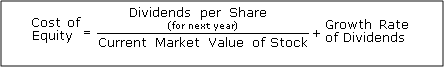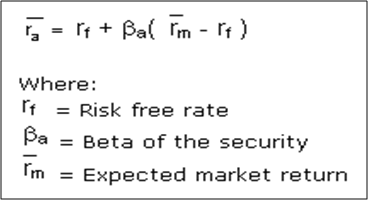What do we understand by cost of equity why it is not dividends - Motilal Oswal

# What do we understand by cost of equity - why it is not dividendsYou must have heard of the term cost of equity quite often. What do we understand by cost of equity and what is meant by cost of equity. The easiest explanation of cost of equity is the dividend cost. There are investors who argue that the only cost that the company incurs when it issues equity shares is the dividend that it pays out to the shareholders. But, there is a problem with this argument. There are companies that do not pay dividends either because they are making a loss or because they are ploughing back profits. Does that mean that these companies have zero cost of equity? That is obviously an erroneous definition. Obviously, cost of equity is something bigger.

Broadly, there are two approaches to calculating the cost of equity. The first approach uses the cost of equity formula dividend growth model as the basis for calculating cost of equity. What this model assumes is that the investors do invest in equities for the sake of consistent payment of dividends. So cost of equity can be looked at as a combination of the current dividend yield and the expected growth in dividend. The second approach is more scientific and is also more accepted as a global measure of cost of equity. It uses the Capital Asset Pricing Model (CAPM) approach which assumes that greater the risk in a stock greater is the return that shareholders expect and therefore greater the cost of equity for such stocks. This is intuitively correct because when you buy a mid-cap or small share you expect to earn a higher return compared to a large cap stock. Let us now understand the two approaches to calculating the cost of equity and how to calculate the cost of equity in practice.

Using the Dividend Growth model for cost of equity
Let us first look at the formula that we can use to calculate the cost of equity using the dividend growth model..If you observe the above formula, there are 2 aspects to the cost of equity as per the dividend growth model. The first part of the formula is the dividend yield and the second part of the formula is the Growth rate in dividends. For example if the dividend yield is 5% and the growth rate of dividends on a sustainable basis is 7% then the cost of equity can be assumed to be 12% in the above case. This formula can work for stable industries where the dividend policy has stabilized. However, for sectors like telecom or power with long gestation periods and low dividend yields, this formula can skew the picture of cost of capital. In such cases, the CAPM will provide a better picture of the cost of equity.

Cost of equity using the CAPM method
This method of calculating cost of equity is not only more sophisticated but also more practical and hence used more widely. The CAPM looks at the cost of equity as the required rate of return for investors. Normally, higher the risk perceived in a stock higher is the return expectation. That will obviously mean that such companies will have a higher cost of equity (meaning they need to satiate a higher return requirement for shareholders). The formula for CAPM looks somewhat like this..The required rate of return for shareholders (cost of equity) as per the CAPM is a function of 3 factors..

Risk free rate of returns is the return that you would earn on the most risk free instrument in the economy like a government bond which has no default risk. Obviously, that will be the base as if you are not even going to earn that then you are better off invested in safe government bonds.

Rm represents the market rate of return or what you can earn on the index like Nifty or Sensex over longer periods of time. When you invest in equities, you are taking a higher risk than bond buyers. So you expect a premium over what you can earn on bonds. (Rm – Rf) represents the market premium that investors expect when they take on the higher risk of equities.

Beta is, probably, the most significant aspect of the cost of equity calculation. Beta shows whether a stock is aggressive or defensive. A stock with a Beta of greater than 1 is considered to be aggressive and a stock with a Beta of less than 1 is considered to be defensive. Beta measures the risk that you cannot diversify away and a stock with a Beta of 1.2 is more risky than a stock with a Beta of 0.9. So, higher the Beta higher is the compensation that shareholders expect from the stock. Compensation for Beta is necessary because in the absence of that compensation investors have no incentive to invest in stocks and they will just invest in index funds and be happy with market returns.

CAPM brings about a relationship between risk and return expected
The reason the CAPM model for cost of equity is more practical is that it brings about a direct relationship between risk and the expected rate of return. It assumes that all the unsystematic risk is diversified and only the systematic risk measured by Beta remains. So it is only for the Beta that shareholders expect compensation. This model is still not perfect when it comes to calculating cost of equity, but it offers a close approximation of reality!

• Open your FREE Demat Account in 5 Minutes
+91|
Select State
Select City
By submitting your details, you are authorising us to call you or send promotional communication even though you may be registered under DND